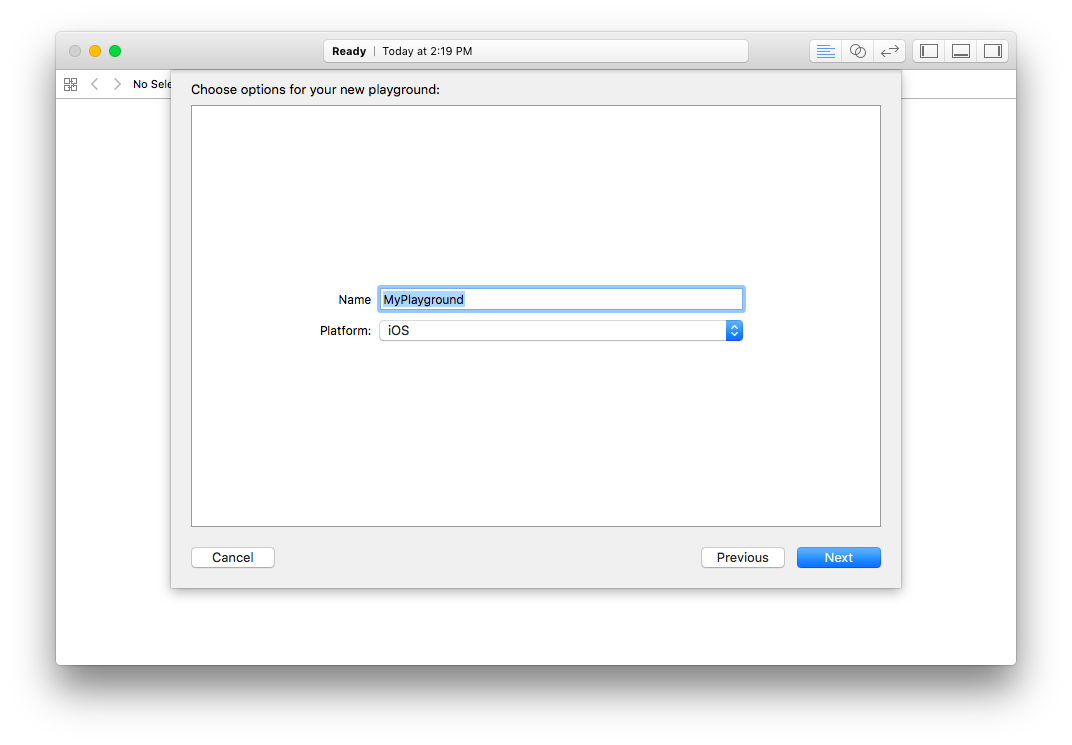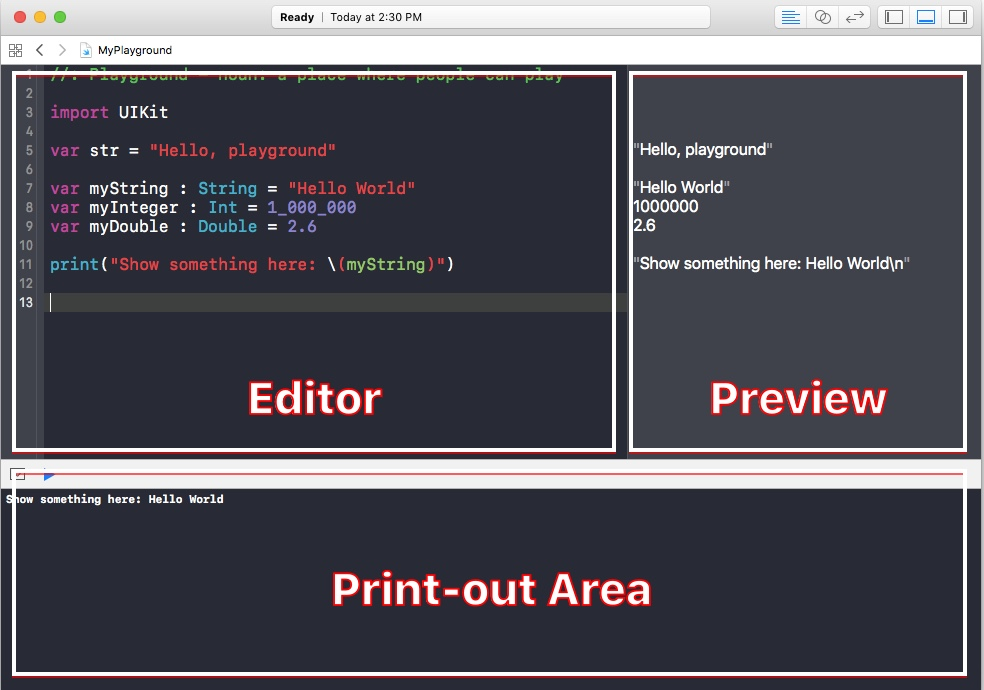# Learn Swift 3 + Xcode Playground

5 minutes, 10 seconds

You will need Xcode 8, and we will write Swift 3.

Coding is key for education in information age. In the future many jobs will be replaced by computers. And what human will do, coding the computer to replace their job.

Why do we need computer to replace our job? Computer can do the same task over and over again without doing mistakes or feeling bored. But first we have to teach computer to what to do.

Today we will be learning to use Xcode Playground to learn Swift, it eases us to understand coding concept. Unlike traditional way, playground will show the result in-line without having to compile. It suites from just getting started to experienced developers.

Let’s get it started.

## Creating a Swift Playground

To create a new Playground file, open the Xcode. Click File ▶ New ▶ Playground. Save it as ‘MyPlayground’ or any name you like at appropriate place.### Playground User Interface

Playground has 3 areas; editor, preview and print-out section like the image below.When we write codes at editor, preview will show the result when we declare anything. On the Print-out area, it will show when we use `print()` function. He’re is an example code to show on the print-out area.

Let’s try to add some codes that declare somethings. In this case we will declare a String, Int, Double and Bool.

Replace the content inside with this code.

``````
var myString : String = "Hello World"
var myInteger : Int = 1_000_000
var myFloat : Float = 2.6
var myBool : Bool = true
``````

Right after you type the code at editor, immediately you will see on the preview like this after `//`. `//` is a comment-out feature, it will wipe out from computer when reading it. In this case the comment-out works as preview’s result.

``````
var myString : String = "Hello World" // "Hello World"
var myInteger : Int = 1_000_000 // 1000000
var myDouble : Double = 2.6 // 2.6
var myBool : Bool = true // true
``````

The composition of each line you saw, `var` space name that you want to declare followed by colon (`:`) and the type, followed with equal (`=`) and the value on the right hand side.

``````
print("Show something here: \(myString)")
``````

## Maths Operations

One of the thing code can do is mathemathics calculation. It can do addition (+), subtraction (-), multiplication (*) and division (/).

``````
var a : Int = 6
var b : Int = 5
var result : Int = a + b
print ("\(a) + \(b) = \(result)")
// Output
6 + 5 = 11
``````

Subtraction.

``````
// Subtraction
var a2 : Int = 6
var b2 : Int = 5
var result2 : Int = a2 - b2
print ("\(a2) - \(b2) = \(result2)")
// Output
6 - 5 = 1
``````

Multiplication.

``````
// Multiplication
var a3 : Int = 6
var b3 : Int = 5
var result3 : Int = a3 * b3
print ("\(a3) * \(b3) = \(result3)")
// Output
6 * 5 = 30
``````

Division. As we use Int here we will not ever get the decimal point.

``````
// Division
var a4 : Int = 6
var b4 : Int = 5
var result4 : Int = a4 / b4
print ("\(a4) / \(b4) = \(result4)")
// Output
6 / 5 = 1
``````

We however can get the remainder, from it’s division.

``````
// Remainder
var a5 : Int = 6
var b5 : Int = 5
var result5 : Int = a5 % b5
print ("Remainder of \(a5) divided by \(b5) is \(result5)")
// Output
Remainder of 6 divided by 5 is 1
``````

If we want to have decimal point, we have to user other type which is Float. We declare the result as Float as well.

``````
// Division with Float
var a6 : Float = 10.0
var b6 : Float = 3.0
var result6 : Float = a6 / b6
print ("\(a6) / \(b6) = \(result6)")
// Output
10.0 / 3.0 = 3.33333
``````

There is also sibling to Float which is Double. It has double the precesion than Float.

``````
// Division with Double
var a7 : Double = 10.0
var b7 : Double = 3.0
var result7 : Double = a7 / b7
print ("\(a7) / \(b7) = \(result7)")
// Output
10.0 / 3.0 = 3.33333333333333
``````

## Pi (π)

``````
var pi = Float.pi // 3.141593
``````

## Repeat with For Loop

We will make a loop, it works like counting 1 to 10, literally.

``````
for number in 1...10 {
print("\(number)")
}
// Output
1
2
3
4
5
6
7
8
9
10
``````

### Summary

So far we have learnt about Xcode Swift Playground, mathematical operation and also looping. On the next chapter we will see some conditional operation. It will be amazing, see you soon.